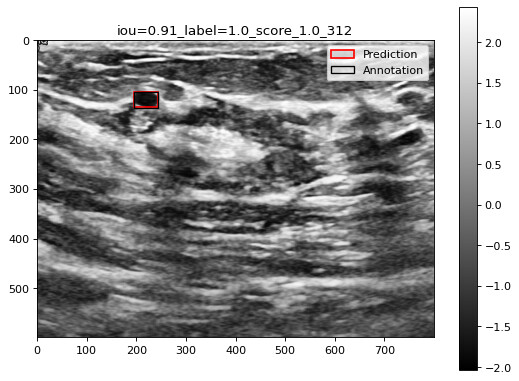# What is the correct Pytorch resnet50 input normalization intensity range

I’m using resnet50 pre-trained as my backbone for faster-rcnn and am trying to normalize the data for fine-tuning. The data original intensity is 0 to 1, then I do some contrast equalization and then convert it back to 0,1 range and apply the Resnet norm (from pytorch page). This results in an odd range (see image below). When I apply a generic normalization (not the resnet preferred) and get it to 0,1 range, the final results are worse after fine-tuning/training.

Should the resnet input images always use the prescribed norm setup and if so, what should the final intensity range be? This looks to be ~zero centered, but would 0,1 provide any advantages. Would there be advantages to normalizing specific to your fine-tuning data instead of using resnet info?``````Pytorch Resnet information:
All pre-trained models expect input images normalized in the same way, i.e. mini-batches of
3-channel RGB images of shape (3 x H x W), where H and W are expected to be at least 224.
The images have to be loaded in to a range of [0, 1] and then normalized using mean =
[0.485, 0.456, 0.406] and std = [0.229, 0.224, 0.225].
``````
``````Generic function used to do the ImageNet norm---
#Imagenet requires a specific Norm to 0,1 then norm with the mean and std from
#the large imagenet dataset
##### NOTE: ToTensor() converts to float32 tensor and adjusts range to 0,1

transform = transforms.Compose([
transforms.ToTensor(),
transforms.Normalize(mean=[0.485, 0.456, 0.406],
std=[0.229, 0.224, 0.225]) ])
``````

I would assume calculating the new dataset stats would be beneficial especially if you are changing the data domain (e.g. from “natural” ImageNet images to medical images).
As a quick check you could calculate the `mean` and `std` of the batches after using the ImageNet `Normalize` and see how strongly it diverges from a zero-mean and unit-variance.

1 Like